# Reasoning Quiz For RRB NTPC : 23rd January 2020 of mirror image, mathematical operation and missing number Series

Q1. If “A” means “subtraction”, “B” means “division”, “C” means “addition” and “D” means “multiplication”, then 305 B 5 A 28 C 43 D 12 = ?
(a) 569
(b) 549
(c) 560
(d) 530

Q2. In the following question, select the missing number from the given series.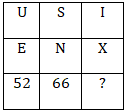(a) 70
(b) 66
(c) 64
(d) 68

Q3. Arrange the given words in the sequence in which they occur in the dictionary.
i. Trinity      ii. Tool
iii. Town      iv. Type
(a) ii, iii, iv, i
(b) i, iii, ii, iv
(c) ii, iii, i, iv
(d) iii, iv, i, ii

Q4. Identify the diagram that best represents the relationship among the given classes.
Balls, Footballs, Spheres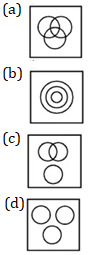Q5. A piece of paper is folded and cut as shown below in the question figures. From the given answer figures, indicate how it will appear when opened.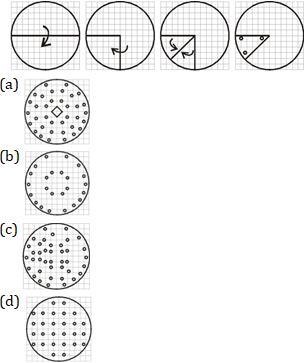Q6. If “A” denotes “added to”, “B” denotes “divided by”, “C” denotes “multiplied by” and “D” denotes “subtracted from”, then 64 B 4 C 4 A 8 D 2 = ?
(a) 65
(b) 75
(c) 70
(d) 80

Q7. If a mirror is placed on the line MN, then which of the answer figures is the right image of the given figure?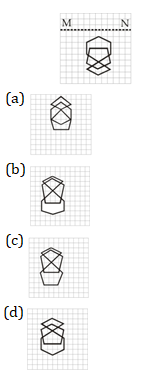Directions(Q8-Q9) Two statements are given followed by two/four conclusions I, II, III and IV. You have to consider the statements to be true even if they seem to be at variance from commonly known facts. You have to decide which of the given conclusions, if any, follow from the given statements.

Q8. Statements: (I) All mugs are tubs.
(II) All tubs are buckets.
Conclusion: (I) All mugs are buckets.
(II) All buckets are mugs.
(a) Conclusion I follows
(b) Conclusion II follows
(c) Neither I nor II follows
(d) Both I and II follow

Q9. Statements: (I) All plates are chairs.
(II) Some chairs are Tables.
Conclusion: (I) All plates are tables.
(II) Some plates are tables.
(a) Conclusion I follows
(b) Conclusion II follows
(c) Neither I nor II follows
(d) Both I and II follows

Q10. Pointing towards a lady, a boy says, “She is the daughter of the woman who is the mother of the husband of my mother”. How is the lady related to the boy ?
(a) Granddaughter
(b) Sister
(c) Cousin
(d) Aunt

Solutions: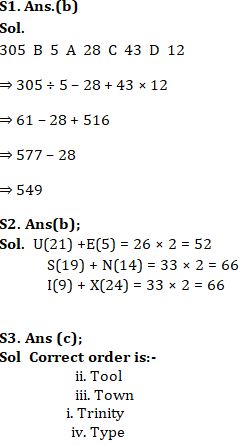S4. Ans(b);
Sol.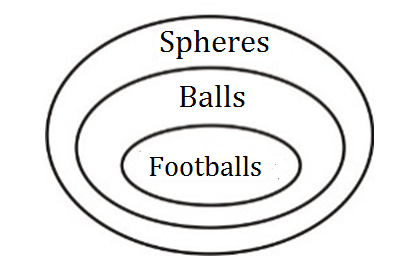S5. Ans(b);

S6. Ans(c);
Sol. 64 B 4 C 4 A 8 D 2
⇒ 64 ÷ 4 × 4 + 8 – 2
⇒ 16 × 4 + 8 – 2
⇒ 64 + 8 – 2
⇒ 72 – 2
⇒ 70
.

S7. Ans(d);

S8. Ans(a);
Sol.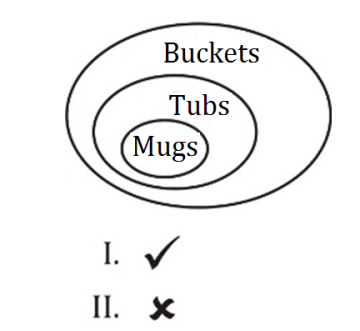S9. Ans(c);
Sol.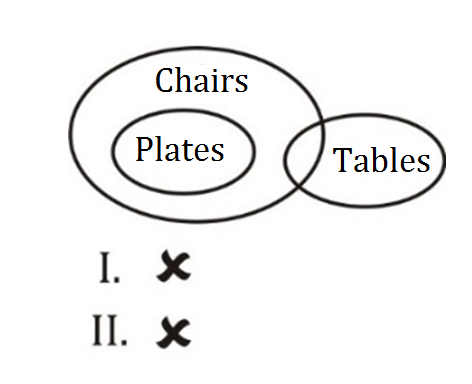Neither I nor II follows.

S10. Ans(d);
Sol.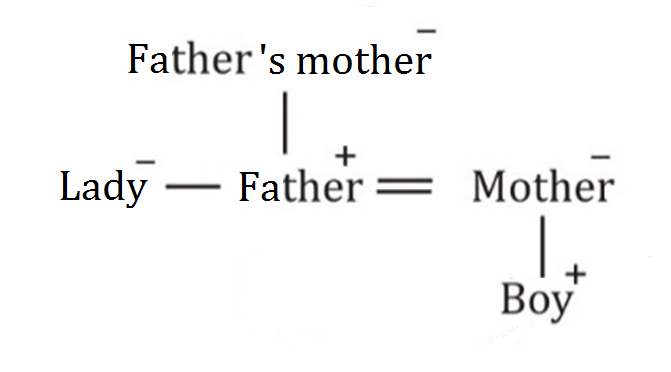Hence, lady is the sister of boy’s father. So. She is his aunt.

##### Reasoning Short Tricks in Hindi For Railway NTPC SSC CHSL CGL Bank & All Exam

Important Links for RRB NTPC Recruitment 2019